Question

# A square, single-turn wire loop ℓ = 1.00 cm on a side is placed inside a...

A square, single-turn wire loop ℓ = 1.00 cm on a side is placed inside a solenoid that has a circular cross section of radius r = 3.00 cm, as shown in the end view of the figure below. The solenoid is 24.0 cm long and wound with 122 turns of wire. (a) If the current in the solenoid is 2.35 A, what is the magnetic flux through the square loop? T · m2 (b) If the current in the solenoid is reduced to zero in 3.45 s, what is the magnitude of the average induced emf in the square loop? V

(a)

l = length of the side of the square = 1 cm = 0.01 m

A = area of the square loop = l2 = 0.01 x 0.01 = 10-4 m2

L = length of the solenoid = 24 cm = 0.24 m

N = number of turns = 122

n = number of turns per unit length = N/L = 122/0.24 = 508.33

i = current in the solenoid = 2.35 A

magnetic field through the solenoid is given as

B =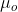ni

magnetic flux though the square loop is given as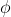= B A=niA

inserting the values= (12.56 x 10-7) (508.33) (2.35) (10-4)= 1.5 x 10-7 Wb

b)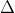i = change in current = 0 - 2.35 = - 2.35 At = time interval = 3.45 sec

Induced emf is given as

E = -nA(i/t )

E = - (12.56 x 10-7) (508.33) (10-4) (- 2.35/3.45 )

E = 4.35 x 10-8 Volts

#### Earn Coins

Coins can be redeemed for fabulous gifts.

Similar Homework Help Questions
• ### A square, single-turn wire loop ℓ = 1.00 cm on a side is placed inside a...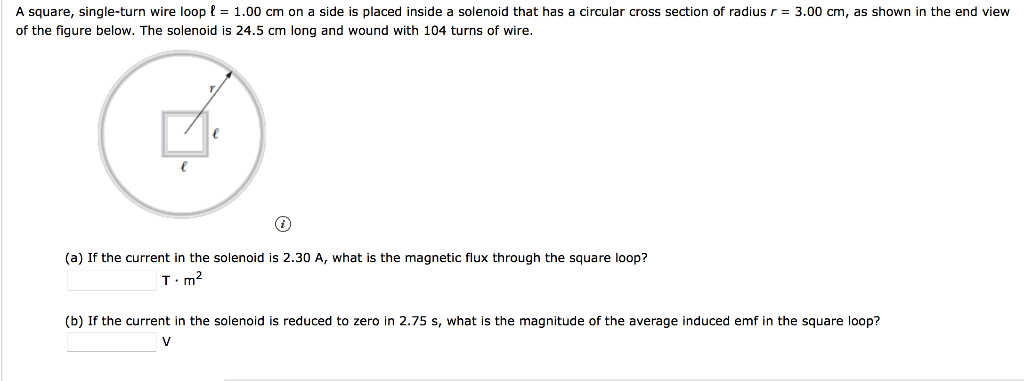A square, single-turn wire loop ℓ = 1.00 cm on a side is placed inside a solenoid that has a circular cross section of radius r = 3.00 cm, as shown in the end view of the figure below. The solenoid is 24.5 cm long and wound with 104 turns of wire. (a) If the current in the solenoid is 2.30 A, what is the magnetic flux through the square loop? T · m2 (b) If the current in the...

• ### A square, single-turn wire loop1.00 cm on a side is placed inside a solenoid is 23.0...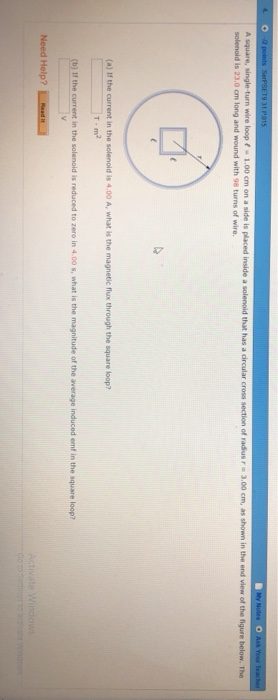A square, single-turn wire loop1.00 cm on a side is placed inside a solenoid is 23.0 cm long and wound with 98 turns of wire that has a circular cross of radius r 3.00 cm, as shown in the end view of the figure below. The (a) If the current in the solenoid is 4.00 A, what is the magnetic flux through the square loop? T-m2 lǐ the current in the solen old is reduced to zero in 4.00 s,...

• ### A square, single-turn wire loop ! - 1.00 cm on a side is placed inside a...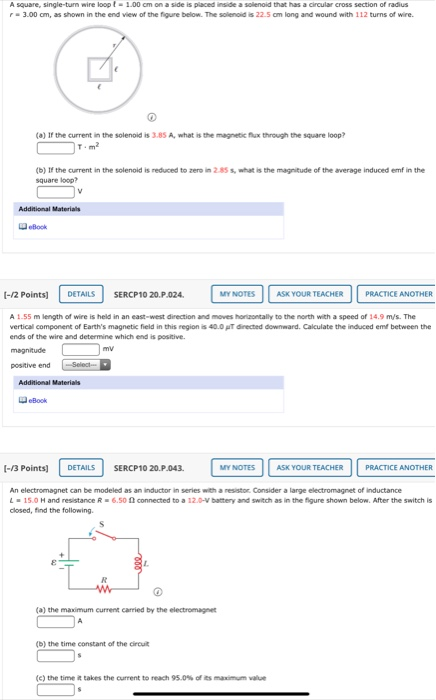A square, single-turn wire loop ! - 1.00 cm on a side is placed inside a solenoid that has a circular cross section of radius - 3.00 cm, as shown in the end view of the figure below. The solenoid is 22.5 cm long and wound with 112 turns of wire. (a) If the current in the solenoid is 3.85 A, what is the magnetic flux through the square loop? (b) If the current in the solenoid is reduced to...

• ### A square, single-turn wire coil L = 1.52 cm on a side is placedinside a...

A square, single-turn wire coil L = 1.52 cm on a side is placed inside a solenoid that has a circular cross-section of radius r = 2.79 cm, as shown in the figure below.The solenoid is 18.0 cm long and wound with 107 turns of wire. If the current in the solenoid is 3.38 A, find the flux through the coil.If the current in the solenoid is reduced to zero in 3.37 s, calculate the magnitude of the average induced...

• ### A square loop with a single turn, with side L= 0.45cm is placed inside a solenoid...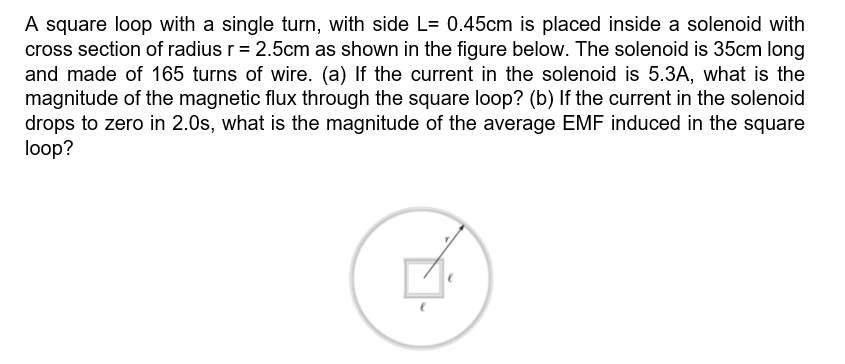A square loop with a single turn, with side L= 0.45cm is placed inside a solenoid with cross section of radius r = 2.5cm as shown in the figure below. The solenoid is 35cm long and made of 165 turns of wire. (a) If the current in the solenoid is 5.3A, what is the magnitude of the magnetic flux through the square loop? (b) If the current in the solenoid drops to zero in 2.0s, what is the magnitude of...

• ### A square loop of wire with a small resistance is moved with constant speed from a...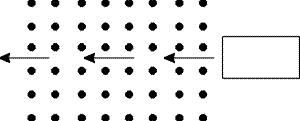A square loop of wire with a small resistance is moved with constant speed from a field free region into a region of uniform B field (B is constant in time) and then back into a field free region to the left. The self inductance of the loop is negligible. When entering the field the coil experiences a magnetic force to the left. When leaving the field the coil experiences a magnetic force to the right. Upon entering the field,...

• ### Square Coil, Round Hole Due this Friday, Feb 23 at 11:59 pm (EST) A square, single-turn...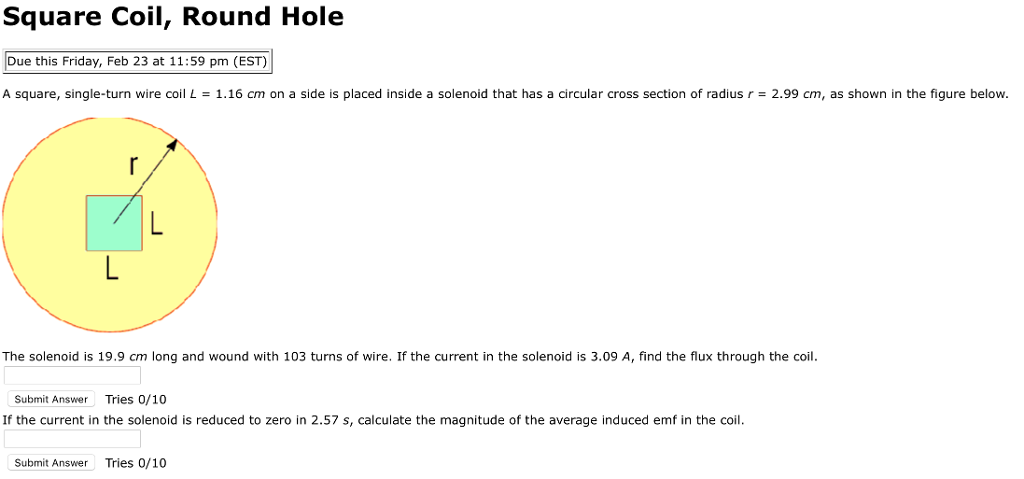Square Coil, Round Hole Due this Friday, Feb 23 at 11:59 pm (EST) A square, single-turn wire coil L = 1.16 cm on a side is placed inside a solenoid that has a circular cross section of radius r = 2.99 cm, as shown in the figure below The solenoid is 19.9 cm long and wound with 103 turns of wire. If the current in the solenoid is 3.09 A, find the flux through the coil Submit Answer Tries 0/10...

• ### Review: Square Coil, Round Hole Due this Friday, Mar 29 at 11:59 pm (EDT) A square,...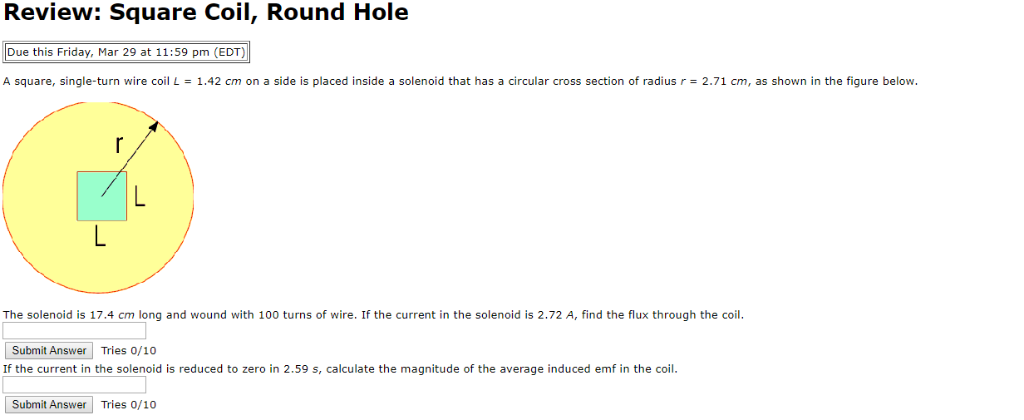Review: Square Coil, Round Hole Due this Friday, Mar 29 at 11:59 pm (EDT) A square, single-turn wire coil L-1.42 cm on a side is placed inside a solenoid that has a circular cross section of radius r 2.71 cm, as shown in the figure below. The solenoid is 17.4 cm long and wound with 100 turns of wire. If the current in the solenoid is 2.72 A, find the flux through the coil Submit Answer Tries 0/10 If the...

• ### A square conducting loop with side length a = 1.25 cm is placed at the center...

A square conducting loop with side length a = 1.25 cm is placed at the center of a solenoid 40.0 cm long with a current of 4.60 A flowing through its 470 turns, and it is aligned so that the plane of the loop is perpendicular to the long axis of the solenoid. The radius of the solenoid is 5.00 cm. (a) What is the magnetic flux through the loop? Wb (b) What is the magnitude of the average emf...

• ### Please fast 4. A single-turn square loop of wire, 2.00 cm on each edge, carries a...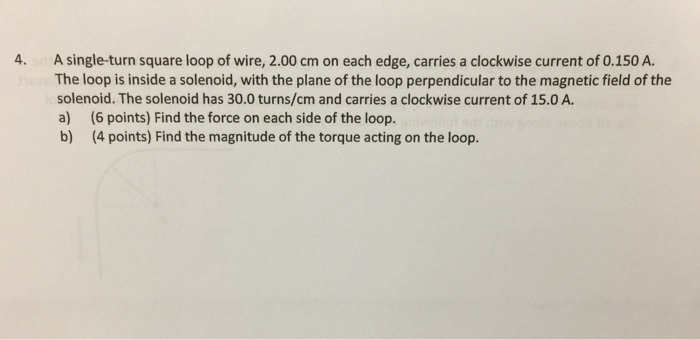Please fast 4. A single-turn square loop of wire, 2.00 cm on each edge, carries a clockwise current of 0.150 A The loop is inside a solenoid, with the plane of the loop perpendicular to the magnetic field of the solenoid. The solenoid has 30.0 turns/cm and carries a clockwise current of 15.0 A a) (6 points) Find the force on each side of the loop b) (4 points) Find the magnitude of the torque acting on the loop.